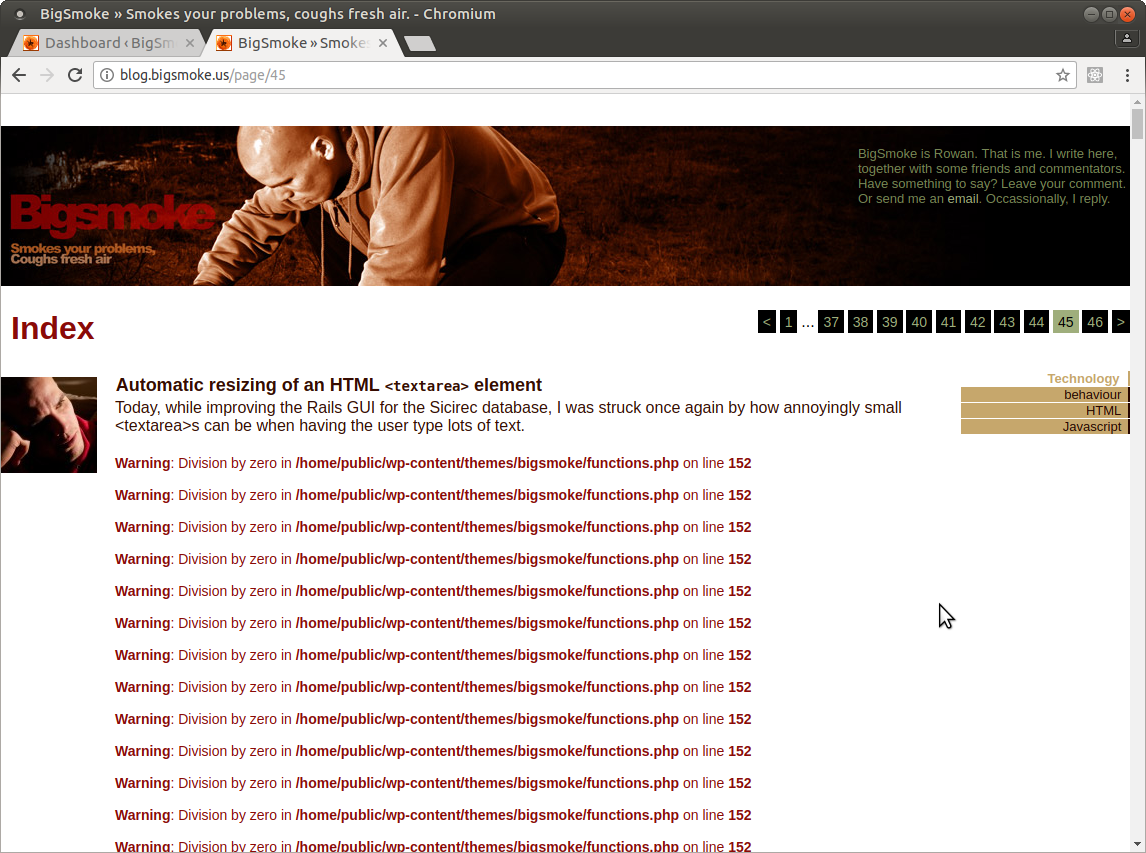I just noticed that none of my posts older than a decade could be listed:The culprit was in the following function, where I had to add "century" and "10" to the lists of \$periods and \$lengths respectively.

```function bigsmoke_ago(\$timestamp_gmt)
{
\$difference = current_time('timestamp', true) - \$timestamp_gmt;
\$periods = array("second", "minute", "hour", "day", "week", "month", "year", "decade");
\$lengths = array("60","60","24","7","4.35","12","10");

for(\$j = 0; \$difference >= \$lengths[\$j]; \$j++)
\$difference /= \$lengths[\$j];
\$difference = round(\$difference);

if(\$difference != 1) \$periods[\$j].= "s";
\$text = "\$difference \$periods[\$j] ago";

if (\$j < 3) {
\$current_day = date('j', current_time('timestamp', true));
\$arg_day = date('j', \$timestamp_gmt);
\$relative_day = (\$current_day == \$arg_day ? 'today' : 'yesterday');

\$text = "\$relative_day, \$text";
}

return \$text;
}```